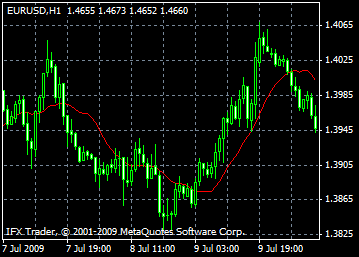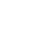## 即时开户InstaForex奖金

# 移动平均指标：描述，设置和应用: 介绍、调整和应用

#### 移动平均线的类型：#### 计算移动平均线的公式：

SMA = 总和 (收盘, N)/N

SUM — 总和;

N — 周期数。

EMA = (收盘 (i)*P) + (EMA (i-1)*(100-P))

EMA (i-1) — 前期的MA读数;

P — 使用价格读数的百分比。

SUM1 = SUM(收盘, N)

SMMA1 = SUM1/N

SMMA (i) = (SUM1-SMMA1+收盘 (i))/N

SUM — 总和;

SUM1 — 从前一栏开始的N个期间的收盘价总和;

SMMA (i - 1) — 前一栏的平滑移动平均线;

SMMA (i) — 当前栏的平滑移动平均线（第一个除外）;

N — 平滑的周期。

LWMA = SUM (收盘 (i)*i, N)/SUM (i, N)

SUM — 总和;

SUM (i, N) — 权重系数的总和;

N — 平滑的周期。• English
• Русский
• العربية
• Bahasa Indonesia
• Bahasa Melayu
• বাংলা
• Български
• 中文
• Español
• हिन्दी
• Asụsụ Igbo
• Português
• اردو
• ไทย
• Українська
• Tiếng Việt
• Èdè Yorùbá